Borůvka's Algorithm in Python - Theory and Implementation - Stack Abuse

# Borůvka's Algorithm in Python - Theory and Implementation### Introduction

Borůvka's Algorithm is a greedy algorithm published by Otakar Borůvka, a Czech mathematician best known for his work in graph theory. Its most famous application helps us find the minimum spanning tree in a graph.

A thing worth noting about this algorithm is that it's the oldest minimum spanning tree algorithm, on record. Borůvka came up with it in 1926, before computers as we know them today even existed. It was published as a method of constructing an efficient electricity network.

In this guide, we'll take a refresher on graphs, and what minimum spanning trees are, and then jump into Borůvka's algorithm and implement it in Python:

### Graphs and Minimum Spanning Trees

A graph is a abstract structure that represents a group of certain objects called nodes (also known as vertices), in which certain pairs of those nodes are connected or related. Each one of these connections is called an edge.

A tree is an example of a graph: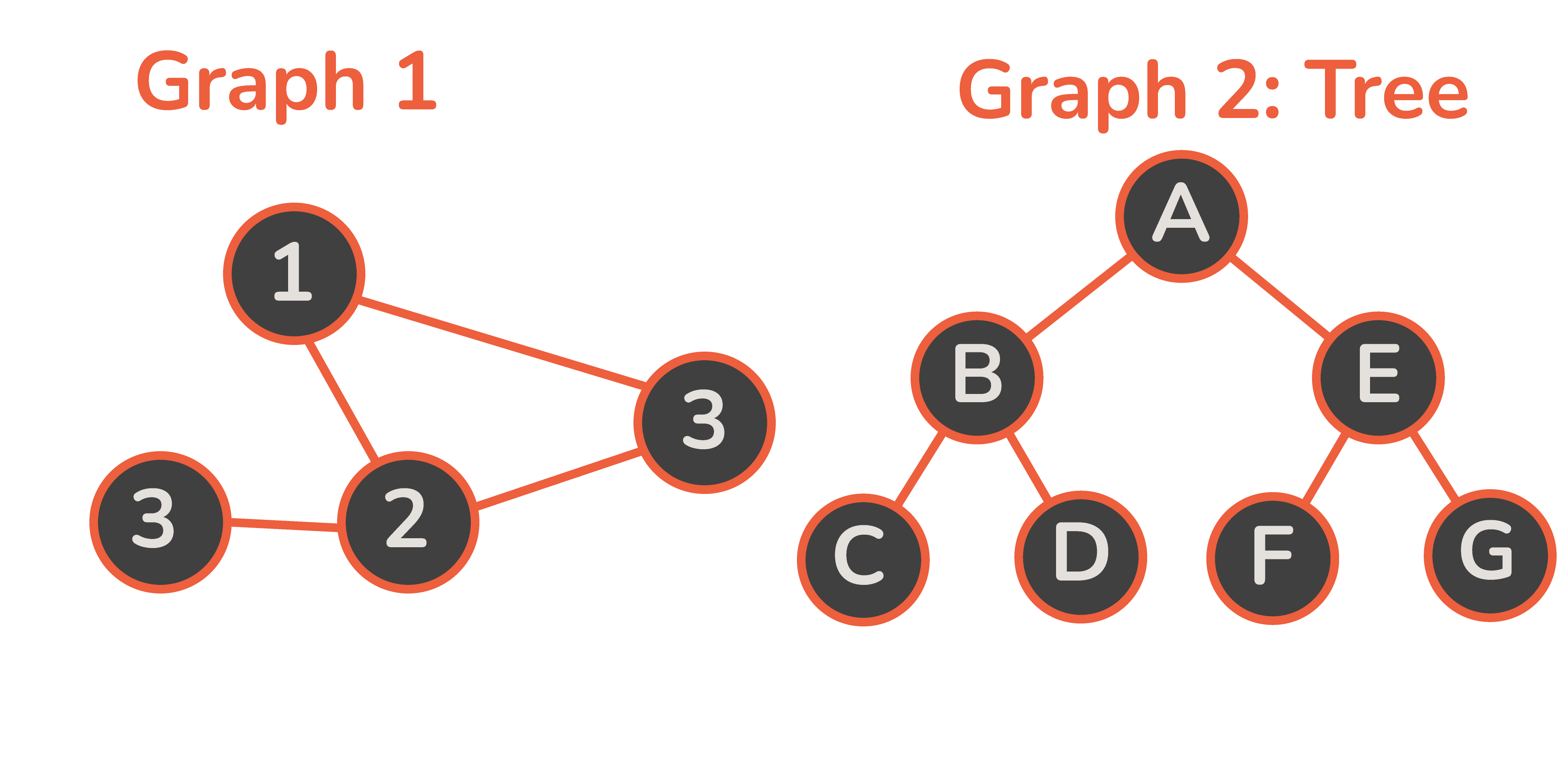In the image above, the first graphs has 4 nodes and 4 edges, while the second graph (a binary tree) has 7 nodes and 6 edges.

Graphs can be applied to many problems, from geospatial locations to social network graphs and neural networks. Conceptually, graphs like these are all around us. For example, say we'd like to plot a family tree, or explain to someone how we met our significant other. We might introduce a large number of people and their relationships to make the story as interesting to the listener as it was to us.

Since this is really just a graph of people (nodes) and their relationships (edges) - graphs are a great way to visualize this: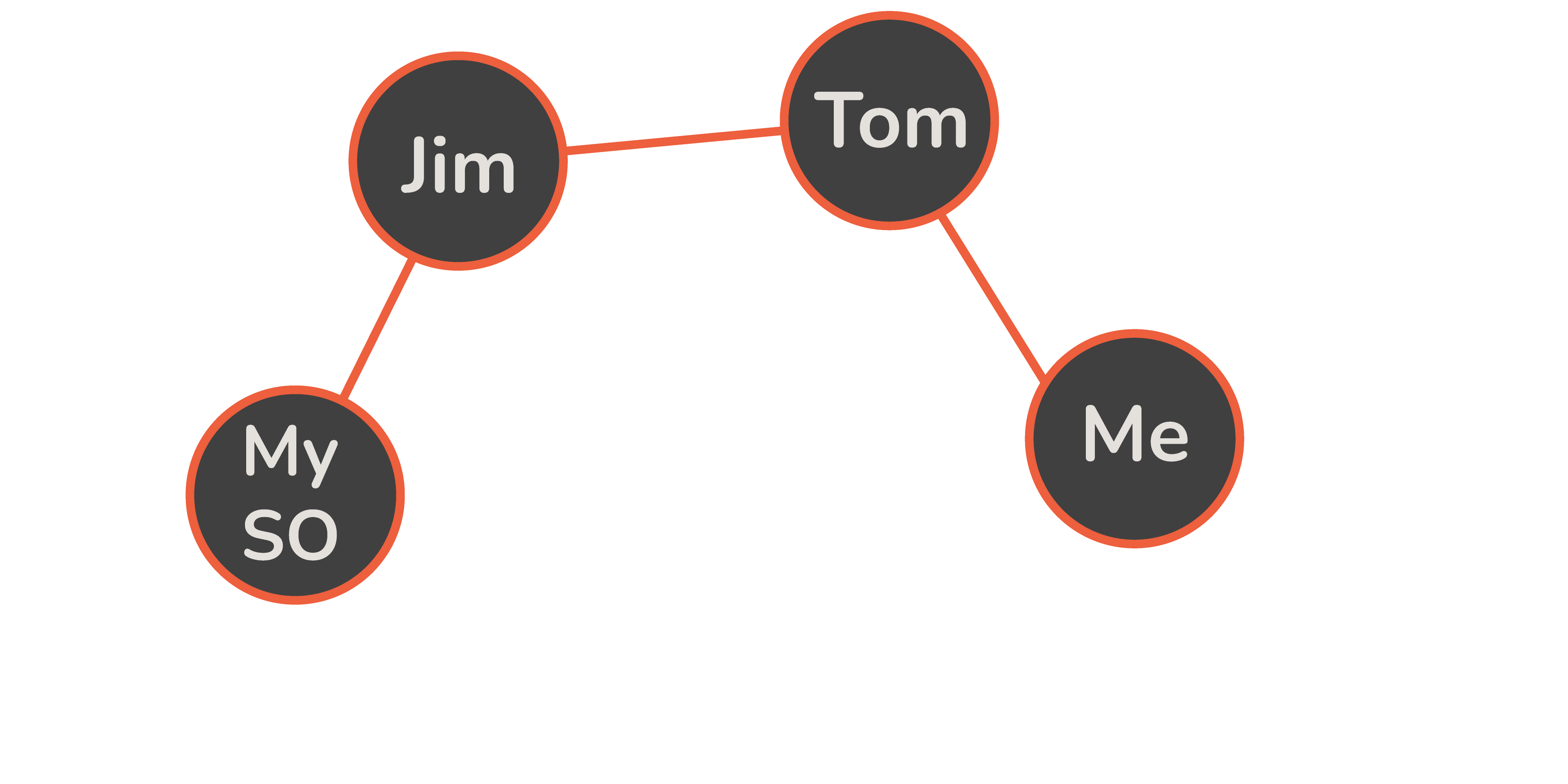#### Types of Graphs

Depending on the types of edges a graph has, we have two distinct categories of graphs:

• Undirected graphs
• Directed graphs

An undirected graph is a graph in which the edges do not have orientations. All edges in an undirected graph are, therefore, considered bidirectional.

Formally, we can define an undirected graph as G = (V, E) where V is the set of all the graph's nodes, and E is a set that contains unordered pairs of elements from E, which represent edges.

Unordered pairs here means that the relationship between two nodes is always two-sided, so if we know there's an edge that goes from A to B, we know for sure that there's an edge that goes from B to A.

A directed graph is a graph in which the edges have orientations.

Formally, we can define a directed graph as G = (V, E) where V s the set of all the graph's nodes, and E is a set that contains ordered pairs of elements from E.

Ordered pairs imply that the relationship between two nodes can be either one or two-sided. Meaning that if there's an edge that goes from A to B, we can't know if there's an edge that goes from B to A.

The direction of an edge is denoted with an arrow. Keep in mind that two-sided relationships can be shown either by drawing two distinct arrows or just drawing two arrow points on either side of the same edge:Another way to differentiate graphs based on their edges is regarding the weight of those edges. Based on that, a graph can be:

• Weighted
• Unweighted

A weighted graph is a graph in which every edge is assigned a number - its weight. These weights can represent the distance between nodes, capacity, price et cetera, depending on the problem we're solving.

Weighted graphs are used pretty often, for example in problems where we need to find the shortest or, as we will soon see, in problems in which we have to find a minimum spanning tree.

An unweighted graph does not have weights on its edges.

Note: In this article, we will focus on undirected, weighted graphs.

A graph can also be connected and disconnected. A graph is connected if there is a path (which consists of one or more edges) between each pair of nodes. On the other hand, a graphs is disconnected if there is a pair of nodes which can't aren't connected by a path of edges.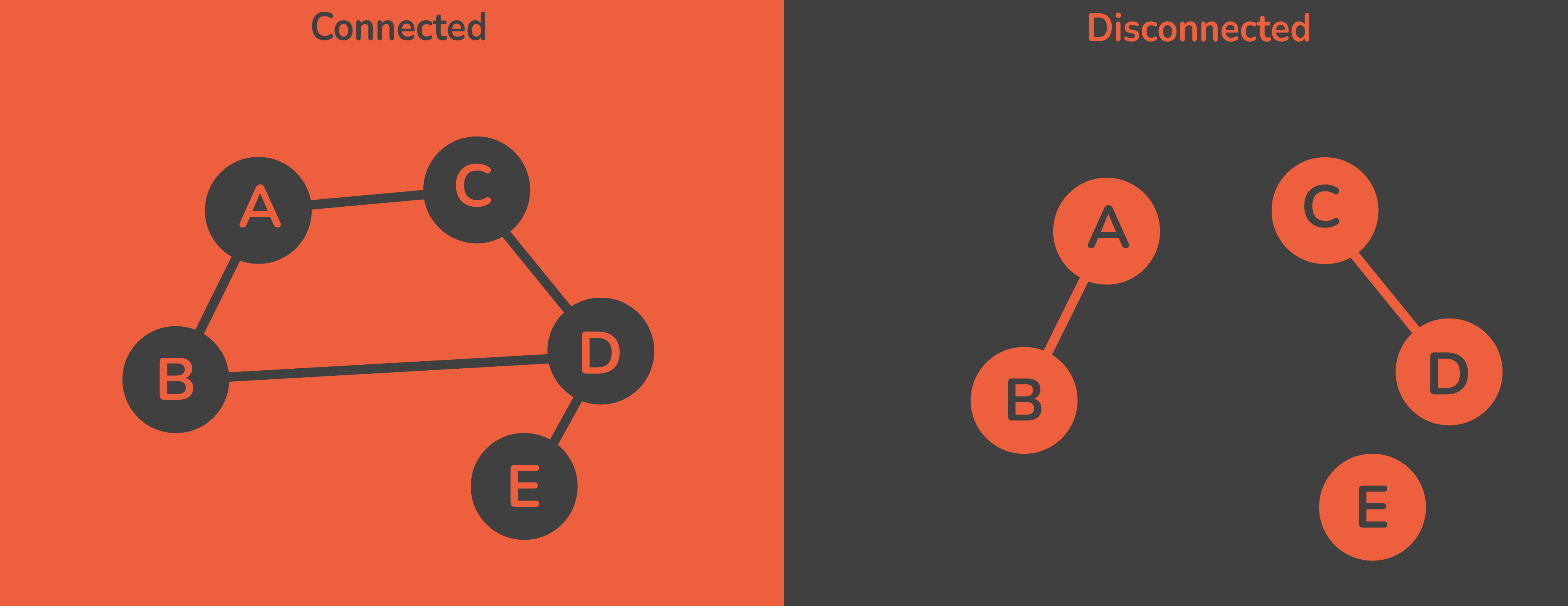#### Trees and Minimum Spanning Trees

There's a fair bit to be said about trees, subgraphs and spanning trees, though here's a really quick and concise breakdown:

• A tree is an undirected graph where each two nodes have exactly one path connecting them, no more, no less.

• A subgraph of a graph A is a graph that is compromised of a subset of graph A's nodes and edges.

• A spanning tree of graph A is a subgraph of graph A that is a tree, whose set of nodes is the same as graph A's.

• A minimum spanning tree is a spanning tree, such that the sum of all the weights of the edges is the smallest possible. Since it's a tree (and the edge weight sum should be minimal), there shouldn't be any cycles.

Note: In case all edge weights in a graph are distinct, the minimum spanning tree of that graph is going to be unique. However, if the edge weights are not distinct, there can be multiple minimum spanning trees for only one graph.

Now that we're covered in terms of graph theory, we can tackle the algorithm itself.

### Borůvka's Algorithm

The idea behind this algorithm is pretty simple and intuitive. We mentioned before that this was a greedy algorithm.

When an algorithm is greedy, it constructs a globally "optimal" solution using smaller, locally optimal solutions for smaller subproblems. Usually, it converges with a good-enough solution, since following local optimums doesn't guarantee a globally optimum solution.

Simply put, greedy algorithms make the optimal choice (out of currently known choices) at each step of the problem, aiming to get to the overall most optimal solution when all of the smaller steps add up.

You could think of greedy algorithms as a musician who's improvising at a concert and will in every moment play what sounds the best. On the other hand, non-greedy algorithms are more like a composer, who'll think about the piece they're about to perform, and take their time to write it out as sheet music.

Now, we will break down the algorithm in a couple of steps:

1. We initialize all nodes as individual components.
2. We initialize the minimum spanning tree S as an empty set that'll contain the solution.
3. If there is more than one component:
• Find the minimum-weight edge that connects this component to any other component.
• If this edge isn't in the minimum spanning tree S, we add it.
4. If there is only one component left, we have reached the end of the tree.

This algorithm takes a connected, weighted and undirected graph as an input, and its output is the graph's corresponding minimum spanning tree.

Let's take a look at the following graph and find its minimum spanning tree using Borůvka's algorithm:At the start, every represents an individual component. That means that we will have 9 components. Let's see what the smallest weight edges that connect these components to any other component would be:

Component Smallest weight edge that connects it to some other component Weight of the edge
{0} 0 - 1 4
{1} 0 - 1 4
{2} 2 - 4 2
{3} 3 - 5 5
{4} 4 - 7 1
{5} 3 - 5 10
{6} 6 - 7 1
{7} 4 - 7 1
{8} 7 - 8 3
Now, our graph is going to be in this state: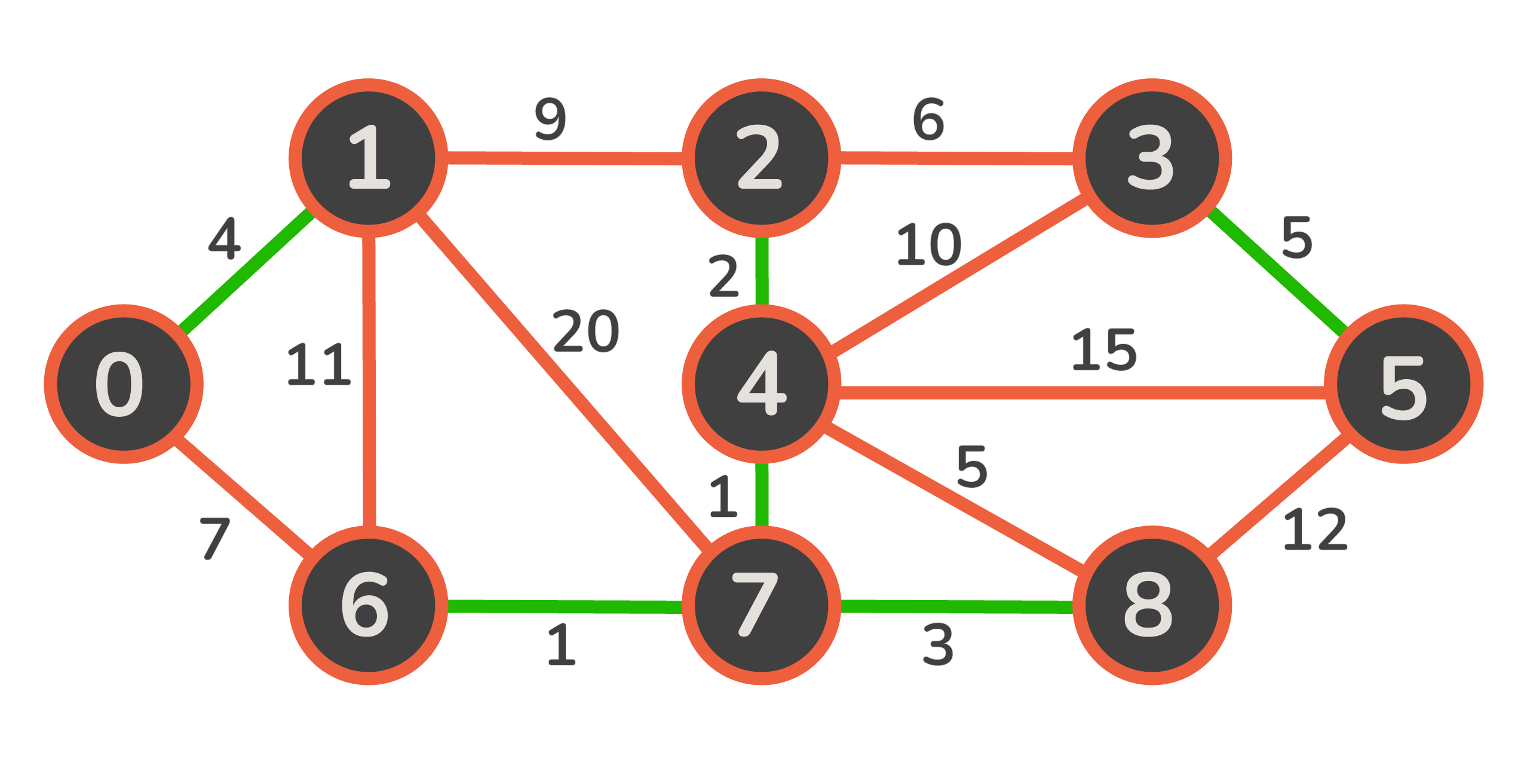## Better understand your data with visualizations.

•  30-day no-questions money-back guarantee
•  Beginner to Advanced
•  Updated regularly (latest update June 2021)
•  Updated with bonus resources and guides

The green edges in this graph represent the edges that bind together its closest components. As we can see, now we have three components: {0, 1}, {2, 4, 6, 7, 8} and {3, 5}. We repeat the algorithm and try to find the minimum-weight edges that can bind together these components:

Component Smallest weight edge that connects it to some other component Weight of the edge
{0, 1} 0 - 6 7
{2, 4, 6, 7, 8} 2 - 3 6
{3, 5} 2 - 3 6

Now, our graph is going to be in this state: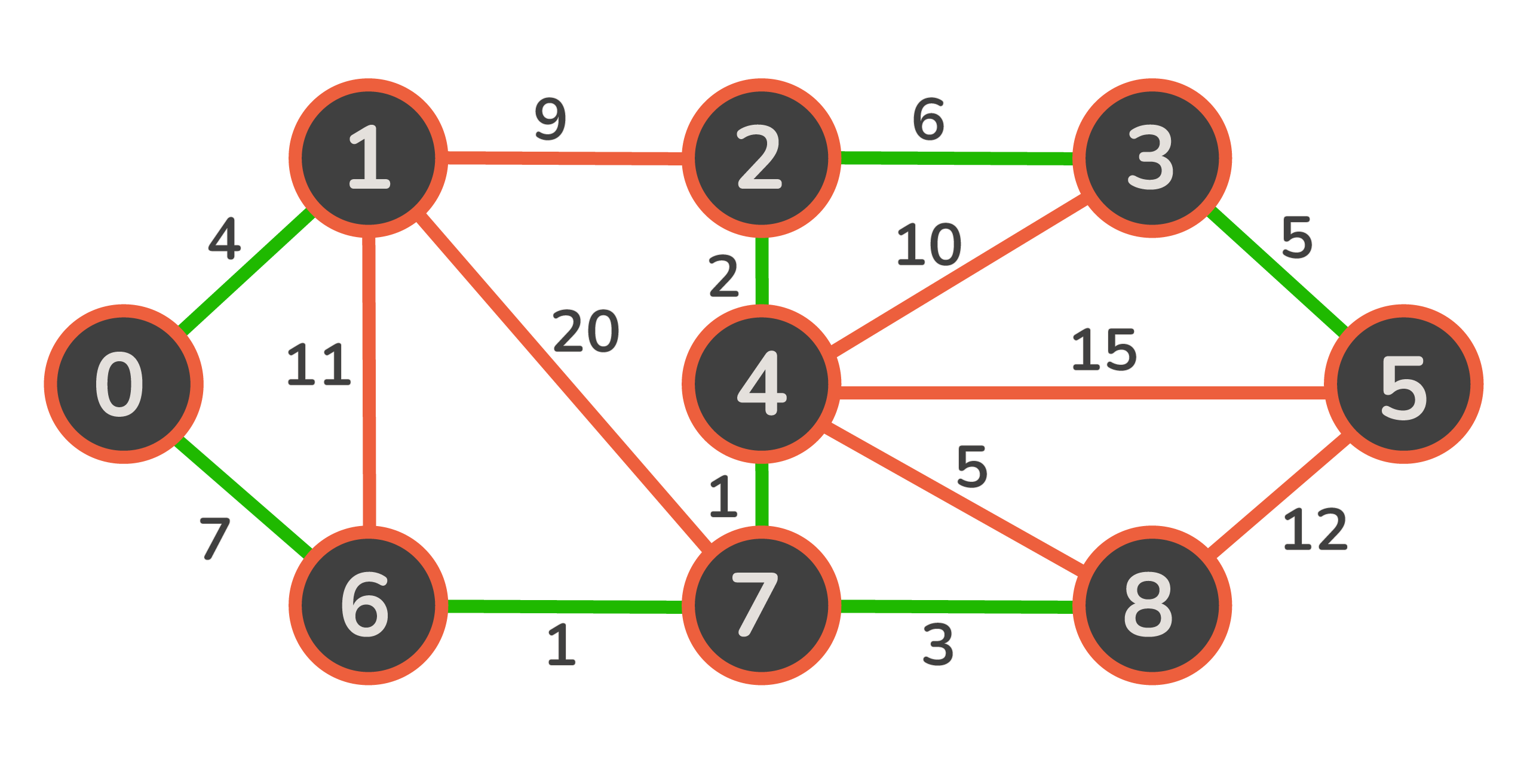As we can see, we are left with only one component in this graph, which represents our minimum spanning tree! The weight of this tree is 29, which we got after summing all of the edges: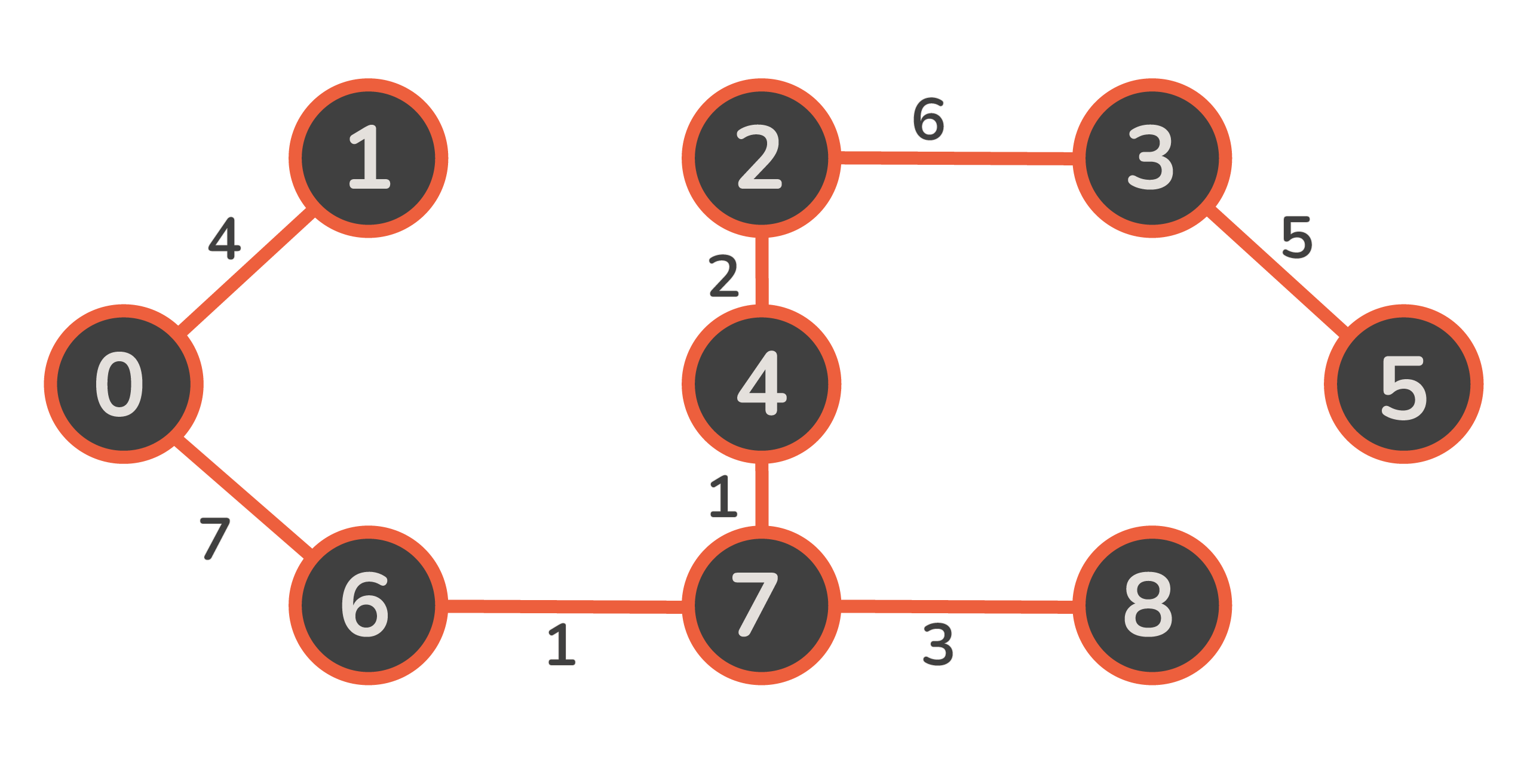Now, the only thing left to do is implement this algorithm in Python.

## Implementation

We are going to implement a Graph class, which will be the main data structure we'll be working with. Let's start off with the constructor:

class Graph:
def __init__(self, num_of_nodes):
self.m_v = num_of_nodes
self.m_edges = []
self.m_component = {}


In this constructor, we provided the number of nodes in the graph as an argument, and we initialized three fields:

• m_v - the number of nodes in the graph.
• m_edges - the list of edges.
• m_component - the dictionary which stores the index of the component which a node belongs to.

Now, let's make a helper function that we can use to add an edge to a graph's nodes:

    def add_edge(self, u, v, weight):
self.m_edges.append([u, v, weight])


This function is going to add an edge in the format [first, second, edge weight] to our graph.

Because we want to ultimately make a method that unifies two components, we'll first need a method that propagates a new component throughout a given component. And secondly, we'll need a method that finds the component index of a given node:

    def find_component(self, u):
if self.m_component[u] == u:
return u
return self.find_component(self.m_component[u])

def set_component(self, u):
if self.m_component[u] == u:
return
else:
for k in self.m_component.keys():
self.m_component[k] = self.find_component(k)


In this method, we will artificially treat the dictionary as a tree. We ask whether or not we've found the root of our component (because only root components will always point to themselves in the m_component dictionary). If we haven't found the root node, we recursively search the current node's parent.

Note: The reason we don't assume that m_components points to the correct component is because when we start unifying components, the only thing that we know for sure won't change its component index is the root components.

For example, in our graph in the example above, in the first iteration, the dictionary is going to look like this:

index value
0 0
1 1
2 2
3 3
4 4
5 5
6 6
7 7
8 8

We've got 9 components, and each member is the component by itself. In the second iteration, it's going to look like this:

index value
0 0
1 0
2 2
3 3
4 2
5 3
6 7
7 4
8 7

Now, tracing back to the roots, we'll see that our new components will be: {0, 1}, {2, 4, 7, 6, 8} and {3, 5}.

The last method we're going to need before implementing the algorithm itself is the method that unifies two components into one, given two nodes which belong to their respective components:

    def union(self, component_size, u, v):
if component_size[u] <= component_size[v]:
self.m_component[u] = v
component_size[v] += component_size[u]
self.set_component(u)

elif component_size[u] >= component_size[v]:
self.m_component[v] = self.find_component(u)
component_size[u] += component_size[v]
self.set_component(v)

print(self.m_component)


In this function, we find the roots of components for two nodes (which are their component indexes at the same time). Then, we compare the components in terms of size, and attached the smaller one to the larger one. Then, we just add the size of the smaller one to the size of the larger one, because they are now one component.

Finally, if the components are of same size, we just unite them together however we want - in this particular example we did it by adding the second one to the first one.

Now that we've implemented all the utility methods we need, we can finally dive into Borůvka's algorithm:

    def boruvka(self):
component_size = []
mst_weight = 0

minimum_weight_edge = [-1] * self.m_v

for node in range(self.m_v):
self.m_component.update({node: node})
component_size.append(1)

num_of_components = self.m_v

print("---------Forming MST------------")
while num_of_components > 1:
for i in range(len(self.m_edges)):

u = self.m_edges[i]
v = self.m_edges[i]
w = self.m_edges[i]

u_component = self.m_component[u]
v_component = self.m_component[v]

if u_component != v_component:
if minimum_weight_edge[u_component] == -1 or \
minimum_weight_edge[u_component] > w:
minimum_weight_edge[u_component] = [u, v, w]
if minimum_weight_edge[v_component] == -1 or \
minimum_weight_edge[v_component] > w:
minimum_weight_edge[v_component] = [u, v, w]

for node in range(self.m_v):
if minimum_weight_edge[node] != -1:
u = minimum_weight_edge[node]
v = minimum_weight_edge[node]
w = minimum_weight_edge[node]

u_component = self.m_component[u]
v_component = self.m_component[v]

if u_component != v_component:
mst_weight += w
self.union(component_size, u_component, v_component)
print("Added edge [" + str(u) + " - "
+ str(v) + "]\n"
+ "Added weight: " + str(w) + "\n")
num_of_components -= 1

minimum_weight_edge = [-1] * self.m_v
print("----------------------------------")
print("The total weight of the minimal spanning tree is: " + str(mst_weight))


The first thing we did in this algorithm was initialize additional lists we would need in the algorithm:

• A list of components (initialized to all of the nodes).
• A list that keeps their size (initialized to 1), as well as the list of the minimum weight edges (-1 at first, since we don't know what the minimum weight edges are yet).

Then, we go through all of the edges in the graph, and we find the root of components on both sides of those edges.

After that, we are looking for the minimum weight edge that connects these two components using a couple of if clauses:

• If the current minimum weight edge of component u doesn't exist (is -1), or if it's greater than the edge we're observing right now, we will assign the value of the edge we're observing to it.
• If the current minimum weight edge of component v doesn't exist (is -1), or if it's greater than the edge we're observing right now, we will assign the value of the edge we're observing to it.

After we've found the cheapest edges for each component, we add them to the minimum spanning tree, and decrease the number of components accordingly.

Finally, we reset the list of minimum weight edges back to -1, so that we can do all of this again. We keep iterating as long as there are more than one component in the list of components.

Let's put the graph we used in the example above as the input of our implemented algorithm:

g = Graph(9)
g.add_edge(0, 1, 4)
g.add_edge(0, 6, 7)
g.add_edge(1, 6, 11)
g.add_edge(1, 7, 20)
g.add_edge(1, 2, 9)
g.add_edge(2, 3, 6)
g.add_edge(2, 4, 2)
g.add_edge(3, 4, 10)
g.add_edge(3, 5, 5)
g.add_edge(4, 5, 15)
g.add_edge(4, 7, 1)
g.add_edge(4, 8, 5)
g.add_edge(5, 8, 12)


Chucking it in the algorithm's implementation will result in:

---------Forming MST------------
{0: 1, 1: 1, 2: 2, 3: 3, 4: 4, 5: 5, 6: 6, 7: 7, 8: 8}
Added edge [0 - 1]
Added weight: 4

{0: 1, 1: 1, 2: 4, 3: 3, 4: 4, 5: 5, 6: 6, 7: 7, 8: 8}
Added edge [2 - 4]
Added weight: 2

{0: 1, 1: 1, 2: 4, 3: 5, 4: 4, 5: 5, 6: 6, 7: 7, 8: 8}
Added edge [3 - 5]
Added weight: 5

{0: 1, 1: 1, 2: 4, 3: 5, 4: 4, 5: 5, 6: 6, 7: 4, 8: 8}
Added edge [4 - 7]
Added weight: 1

{0: 1, 1: 1, 2: 4, 3: 5, 4: 4, 5: 5, 6: 4, 7: 4, 8: 8}
Added edge [6 - 7]
Added weight: 1

{0: 1, 1: 1, 2: 4, 3: 5, 4: 4, 5: 5, 6: 4, 7: 4, 8: 4}
Added edge [7 - 8]
Added weight: 3

{0: 4, 1: 4, 2: 4, 3: 5, 4: 4, 5: 5, 6: 4, 7: 4, 8: 4}
Added edge [0 - 6]
Added weight: 7

{0: 4, 1: 4, 2: 4, 3: 4, 4: 4, 5: 4, 6: 4, 7: 4, 8: 4}
Added edge [2 - 3]
Added weight: 6

----------------------------------
The total weight of the minimal spanning tree is: 29


The time complexity of this algorithm is O(ElogV), where E represents the number of edges, while V represents the number of nodes.

The space complexity of this algorithm is O(V + E), since we have to keep a couple of lists whose sizes are equal to the number of nodes, as well as keep all the edges of a graph inside of the data structure itself.

### Conclusion

Even though Borůvka's algorithm is not as well known as some other minimum spanning tree algorithms like Prim's or Kruskal's minimum spanning tree algorithms, it gives us pretty much the same result - they all find the minimum spanning tree, and the time complexity is approximately the same.

One advantage that Borůvka's algorithm has compared to the alternatives is that it doesn't need to presort the edges or maintain a priority queue in order to find the minimum spanning tree. Even though that doesn't help its complexity, since it still passes the edges logE times, it is a bit more simple to code.

Last Updated: April 4th, 2021

## Improve your dev skills!

Get tutorials, guides, and dev jobs in your inbox.

No spam ever. Unsubscribe at any time. Read our Privacy Policy.

# Prepping for an interview?

• Improve your skills by solving one coding problem every day
• Get the solutions the next morning via email
• Practice on actual problems asked by top companies, like:

© 2013-2021 Stack Abuse. All rights reserved.